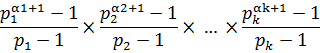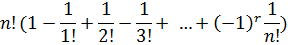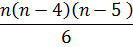Click to Chat

1800-1023-196

+91-120-4616500

CART 0

• 0

MY CART (5)

Use Coupon: CART20 and get 20% off on all online Study Material

ITEM
DETAILS
MRP
DISCOUNT
FINAL PRICE
Total Price: Rs.

There are no items in this cart.
Continue Shopping• Complete JEE Main/Advanced Course and Test Series
• OFFERED PRICE: Rs. 15,900
• View Details

```Revision Notes on Permutations & Combinations

Fundamental Principle of Counting

If one experiment has n possible outcomes and another experiment has m possible outcomes, then there are m × n possible outcomes when both of these experiments are performed simultaneously. In other words, suppose a job has n parts and the job will be completed only when each part is completed. Further, it is known that the first part can be completed in a1 ways, the second part can be completed in a2 ways and so on ...... the nth part can be completed in an ways. Then the total number of ways of doing the job is a1a2a3 ... an. This is also known as the rule of product.

Note:

Fundamental Principal of Counting (FPC) is used to calculate the possibilities of an event without actually counting them.

Basic steps to be used while applying the FPC:

1. Try to identify the independent events involved in a given problem.

2. Find the number of ways of performing or possibilities of occurrence of an event.

3. Multiply these numbers to get the total number of ways of occurrence of these events.

Permutations

The concept of permutation is used for the arrangement of objects in a specific order i.e. whenever the order is important, permutation is used.

The total number of permutations on a set of n distinct objects is given by n! and is denoted as nPn = n!

The total number of permutations on a set of n objects taken r at a time is given by nPr = n!/ (n-r)!

The number of ways of arranging n objects of which r are the same is given by n!/ r!

If we wish to arrange a total of n objects, out of which ‘p’ are of one type, q of second type are alike, and r of a third kind are same, then such a computation is done by n!/p!q!r!

Almost all permutation questions involve putting things in order from a line where the order matters. For example ABC is a different permutation to ACB.

The number of permutations of n distinct objects when a particular object is not to be considered in the arrangement is given by n-1Pr.

The number of permutations of n distinct objects when a specific object is to be always included in the arrangement is given by r.n-1Pr-1.

If we need to compute the number of permutations of n different objects, out of which r have to be selected and each object has the probability of occurring once, twice or thrice… up to r times in any arrangement is given by (n)r.

Circular permutation is used when some arrangement is to be made in the form of a ring or circle.

When ‘n’ different or unlike objects are to be arranged in a ring in such a way that the clockwise and anticlockwise arrangements are different, then the number of such arrangements is given by (n – 1)!

If r things are taken at a time out of n distinct things and arranged along a circle, then the number of ways of doing this is given by nCr(r-1)!.

If clockwise and anti-clockwise are considered to be the same, then the total number of circular permutations is given by (n-1)!/2.

If n persons are to be seated around a round table in such a way that no person has similar neighbor then it is given by ½ (n – 1)!

The number of necklaces formed with n beads of different colors = ½ (n – 1)!

nP0 =1

nP1 = n

nPn = n!/(n-n)! = n! /0! = n!/1 = n!

Combinations

If certain objects are to be arranged in such a way that the order of objects is not important, then the concept of combinations is used.

The number of combinations of n things taken r (0 < r < n) at a time is given by nCr = n!/r!(n-r)!

The relationship between combinations and permutations is nCr = nPr/r!

The number of ways of selecting r objects from n different objects subject to certain condition like:

1. k particular objects are always included =  n-kCr-k

2. k particular objects are never included =  n-kCr

The number of arrangement of n distinct objects taken r at a time so that k particular objects are

(i) Always included = n-kCr-k.r!,

(ii) Never included = n-kCr.r!.

In order to compute the combination of n distinct items taken r at a time wherein, the chances of occurrence of any item are not fixed and may be one, twice, thrice, …. up to r times is given by n+r-1Cr

If there are m men and n women (m > n) and they have to be seated or accommodated in a row in such a way that no two women sit together then total no. of such arrangements = m+1Cn. m! This is also termed as the Gap Method.

If we have n different things taken r at a time in form of a garland or necklace, then the required number of arrangements is given by nCr(r-1)!/2.

If there is a problem that requires n number of persons to be accommodated in such a way that a fixed number say ‘p’ are always together, then that particular set of p persons should be treated as one person. Hence, the total number of people in such a case becomes (n-m+1). Therefore, the total number of possible arrangements is (n-m+1)! m! This is also termed as the String Method.

Let there be n types of objects with each type containing at least r objects. Then the number of ways of arranging r objects in a row is nr.

The number of selections from n different objects, taking at least one =  nC1 + nC2 + nC3 + ... + nCn = 2n - 1.

Total number of selections of zero or more objects from n identical objects is n+1.

Selection when both identical and distinct objects are present:

The number of selections, taking at least one out of a1 + a2 + a3 + ... an + k objects, where a1 are alike (of one kind), a2 are alike (of second kind) and so on ... an are alike (of nth kind), and k are distinct = {[(a1 + 1)(a2 + 1)(a3 + 1) ... (an + 1)]2k} - 1.

Combination of n different things taken some or all of n things at a time is given by 2n – 1.

Combination of n things taken some or all at a time when p of the things are alike of one kind, q of the things are alike and of another kind and r of the things are alike of a third kind = [(p + 1) (q + 1)(r + 1)….] – 1.

The number of ways to select some or all out of (p+q+t) things where p are alike of first kind, q are alike of second kind and the remaining t are different is = (p+1)(q+1)2t – 1.

Combination of selecting s1 things from a set of n1 objects and s2 things from a set of n2 objects where combination of s1 things and s2 things are independent is given by n1Cs1 x n2Cs2

Total number of ways in which n identical items which can be distributed among p persons so that each person may get any number of items is n+p-1Cp-1.

Total number of ways in which n identical items can be distributed among p persons such that each them receive at least one item n-1Cp-1

Some results related to nCr

1. nCr = nCn-r

2. If nCr = nCk, then r = k or n-r = k

3. nCr + nCr-1 = n+1Cr

4. nCr = n/r  n-1Cr-1

5. nCr/nCr-1 = (n-r+1)/ r

6. If n is even nCr is greatest for r = n/2

7. If n is odd, nCr is greatest for r = (n-1)/2,(n+1)/2

Formation of Groups

The number of ways in which (m + n) different things can be divided into two groups, one containing m items and the other containing n items is given by

m+nCn = (m+n)!/ m!n!

In the above case, if m = n i.e. the groups are of same size then the total number of ways of dividing 2n distinct items into two equal groups is given by 2nCn/2!.This can be written as (2n)!/n!n!2!

Remark: The result is divided by 2 in order to avoid repetition i.e. false counting.

The total number of ways of dividing (m + n + p) distinct items into three unequal groups m, n, p is (m + n + p)!/ m!n!p!.

In the above case, if m = n = p, then the total number of ways reduce to (3n)!/(n!)3 3!

The number of ways in which ‘l’ groups of n distinct objects can be formed in such a way that ‘p’ groups are of object n1, q groups of object n2 are given by

n!/ (n1)!p(n2)!q(p!)(q!)

If (a + b + c) distinct items are to be divided into 3 groups and then distributed among three persons, then the number of ways of doing this is

(a + b + c)!. 3!/ a!b!c!

Problems Based on Number Theory

Number of divisors

If N = p1α1 p2α2 ….. pkαk, then

Number of divisors = Number of ways of selecting zero or more objects from the group of identical objects (α1+1)( α2+1)…(αk+1)

This includes 1 and N also.

All divisors excluding 1 and N are called Proper divisors.

Sum of divisors:

If N = p1α1 p2α2 ….. pkαk, then sum of divisors of N is

(1+ p1 + p12 +…+ p1α1) × (1 + p2 + p22 +…+ p2α2) ….. (1 + pk + pk2 +…+ pkαk)

=Number of ways of putting N as a product of two natural numbers is

If n is not a perfect square = ½ (a1 + 1)(a2 + 1) ….. (ak +1)

If n is  a perfect square = ½ [(a1 + 1)(a2 + 1) ….. (ak +1) + 1].

Derangement and Results on Points

If n things are arranged in a row, then the number of ways in which they can be deranged so that r things occupy wrong places while (n-r) things occupy their original places, is

= nCn-r Dr, where

Dr =If n things are arranged in a row, the number of ways of deranging them so that none of them occupies its original place, is

= nC0 Dn

=If there are n points in plane put of which m (< n) are collinear, then the following results hold good:

Total number of different straight lines obtained by joining these n points is

nC2 – mC2 +1

Total number of different triangles formed by joining these n points is nC3 – mC3

Number of diagonals in polygon of n sides is nC2 – n

If m parallel lines in a plane are intersected by a family of other n parallel lines. Then total number of parallelograms so formed is mC2 × nC2

Number of triangles formed by joining vertices of convex polygon of n sides is nC3 of which

Number of triangles having exactly 2 sides common to the polygon = n

Number of triangles having exactly 1 side common to the polygon = n(n-4)

Number of triangles having no side common to the polygon =```### Course Features

• 728 Video Lectures
• Revision Notes
• Previous Year Papers
• Mind Map
• Study Planner
• NCERT Solutions
• Discussion Forum
• Test paper with Video Solution